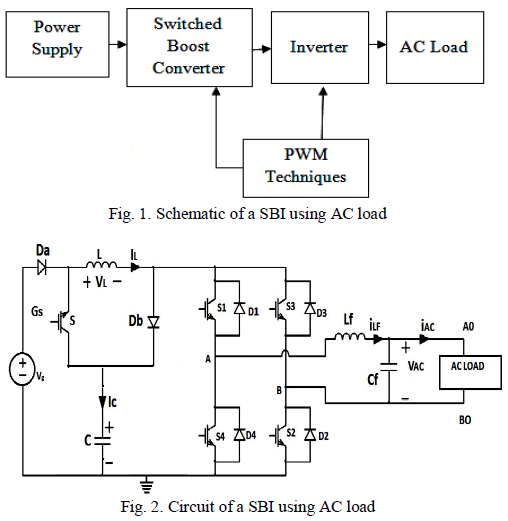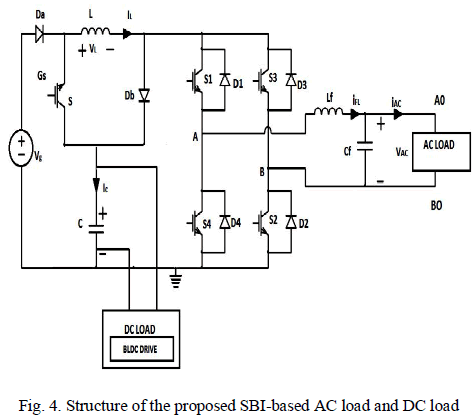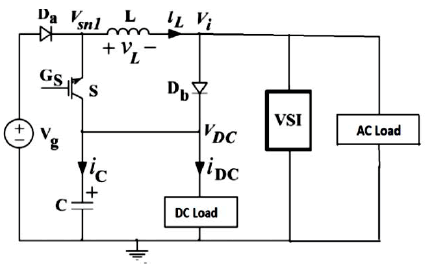ISSN ONLINE(2319-8753)PRINT(2347-6710)

All submissions of the EM system will be redirected to Online Manuscript Submission System. Authors are requested to submit articles directly to Online Manuscript Submission System of respective journal.

# Design and Simulation of Switched Boost Inverter for AC and DC Loads

 Shankar. R, Sankarganesh. R, Ramesh. S Department of EEE, KSR College of Engineering, Tiruchengode, Namakkal, Tamil Nadu, India Related article at Pubmed, Scholar Google

Visit for more related articles at International Journal of Innovative Research in Science, Engineering and Technology

## Abstract

Switched Boost Inverter (SBI) is a single stage DC to AC power converter and it is derived from the Z - source inverter employs an LC impedance network in between the main inverter bridge and the power source. This SBI converter can possible to supply both DC and AC loads simultaneously from a single dc input source. Here, reducing the inverter switching stress. Also, this converter provides to shoot- through of the inverter legs without causing any damage to the inverter switches. The unique feature of the SBI, it can operate either in buck or boost mode operations with a wide range of obtainable output voltages from a given input voltage, unlike the traditional buck-type voltage source inverter.SBI construct similar advantages of converter uses more switching devices and lower number of passive devices compared to the original ZSI. Along with its pulse width modulation (PWM) control strategies is give the inverter switching pulse. These features make the SBI suitable for variable motor speed drive application. The SBI reduce the electromagnetic interference noise immunity when compared to the VSI. which enables the compact design of the power converter.Here BLDC motor drive is using as a DC load and AC load as resistive load and non linear load.The theoretical and modulation concepts presented have been verified in simulation .The operating modes of the proposed converter are simulated using MATLAB.

### Index Terms

pulse width modulation (PWM), switched boost inverter (SBI), AC and DC Loads

### INTRODUCTION

This novel power converter called Switched Boost Inverter (SBI) which works similarly to a Z-source inverter, this topology construct similar properties of a ZSI with minimum number of passive components and many active components. This section presents a review of the approach used to derive the SBI from IWJ topology . The ZSI consists of an X-shaped passive network to couple the main power converter and the power sources. Unlike a traditional VSI ,.the ZSI has the advantage of either stepping up or stepping down the input voltage by properly used the shoot-through state of the inverter bridge which is achieved by allowing the shoot-through of the inverter leg switches. As per the consumer prefers each DC load and AC load in the application also has its own power electronic interface for simplicity.
The application of motor speed control is based on the proposed structure of SBI. The operating principle and Pulse Width Modulation (PWM) control of the SBI has been explained that SBI is a single-stage power converter. That can supply both DC and AC loads simultaneously from a single DC input. So, it can realize both the DC to DC converter and the DC to AC converter in a single stage. So, it has a wide range of obtainable output voltage for a given source voltage.Switched boost inverter has exhibits better Electro Magnetic interferences (EMI) noise immunity when compared to a traditional Voltage source inverter, as the shoot-through (both switches in one leg of the inverter bridge are turned ON or OFF simultaneously) due to EMI noise will not damaged the inverter switches.
This reduces extra burden on the power converter protection circuit and helps in the realization of the compact design of the power converter. As the SBI allows to shoot-through in the inverter legs, it does not require a dead-time circuit and hence, eliminate the need for complex dead-time compensation technologies.
Therefore, this topology may not be suitable for lowpower applications where size, weight, and cost are the main constraints. In this project, the steady-state and smallsignal analyses of the SBI are presented. Also, two different pulse width modulation (PWM) control strategies suitable for the SBI are described . The DC-AC inverters usually operate on Pulse Width Modulation (PWM) technique. The PWM is a very advance and useful technique in which width of the Gate pulses is controlled by various mechanisms. PWM inverter is used to keep the output voltage of the inverter at the rated voltage irrespective of the output load.
The schematic of the SBI in which a switched boost network comprising of one active switch (s),two diodes (Da.Db), one inductor (L), and capacitor(C) is connected between voltage source Vg and the inverter bridge. A low-pass LC filter is used at the output of the inverter bridge to filter the switching frequency components in the inverter output voltage.

### SWITCHED BOOST INVERTER TOPOLOGY FOR AC AND DC LOADSThree different power converter stages are used to interface the renewable energy source, energy storage unit, and the local AC loads in the system to the DC bus. This paper proposes a structure of the variable speed operation using switched boost inverter (SBI) as a power electronic, interface. The structure of the proposed SBI-based variable speed BLDC motor  shows the circuit diagram of the SBI supplying both DC and AC loads.mode I To explain the steady-state operation of the SBI, assume that the inverter is in a shoot-through zero state for duration D. TS in a switching cycle TS. The switch S is also turned on during this interval. As shown in the equivalent circuit of Fig.5(a) the inverter bridge is represented by a short circuit during this interval. The diodes DA and Deb are reverse biased (as VC > VG), and the capacitor C charges the inductor L through switch S and the inverter bridge. The inductor current in this interval equals the capacitor discharging current.
mode II For the remaining duration of the switching cycle (1 −D). TS, the inverter is in non-shoot-through state, and the switch S is turned off. The inverter bridge is represented by a current source in this interval as shown in the equivalent circuit of Fig. 5 (b) now, the voltage source VG and inductor L together supply power to the inverter and the capacitor through diodes DA and DB. The inductor current in this interval equals the capacitor charging current added to the inverter input current. Note that the inductor current is assumed to be sufficient enough for the continuous conduction of diodes DA and DB for the entire interval (1 − D). TS.Fig.6. Connection of a VSI across the switching terminal Vi of the CIWJ topology (switch Si can be realized by using the shoot-through state of the VSI).Using small ripple approximation, (1) –(3) can be rewritten as Note that similar to a ZSI the shoot-through duty ratio(D) of the SBI also cannot exceed 0.5 for a positive DC bus voltage, VDC.
Here, VDC, IL , and IDC are DC components in VDC (t), IL (t), and IDC (t), respectively, and I am the current drawn by inverter bridge in (1 − D) ·TS intervalUnder steady state, the average voltage across the inductor and average current through the capacitor in one switching cycle should be zero. Using volt second balance, we haveSimilarly, using charge-second balance, one can writeThe average DC link voltage VI can be calculated asA. SBI has following advantages:
1)In the event of shoot-through in any phase leg of the inverter bridge, the diode Db is reverse-biased and capacitor C is disconnected from the inverter bridge. Now, the current through the circuit is limited by the inductor L. So, similar to ZSI, shoot-through does not damage the switches of the SBI.
2)As the SBI allows shoot-through, no dead-time is needed to protect the converter. Also this circuit exhibits better EMI noise immunity compared to a VSI.
3) Since dead-time is not required, there is no need of extra dead-time compensation technologies to compensate the waveform distortion caused by dead- time.

### PWM CONTROL OF SBI

The SBI utilizes the shoot-through interval of the Hbridge to invoke the boost operation. So, the traditional PWM techniques of VSI ,  have to be modified to incorporate the shoot-through state, so that they are suitable for SBI. In ,a PWM scheme for SBI is developed based on the traditional sine-triangle PWM with uni polar voltage switching ,,.
This technique has been illustrated in Fig. 7 during positive and negative half cycles of the sinusoidal modulation signal vm(t)
As shown in Fig. 7(b) and (c), the gate control signals GS 1 and GS 2 are generated by comparing the sinusoidal modulation signals vm(t), and –vm(t) shown in Fig. 7(a) with a high-frequency triangular carrier vtri(t) of amplitude Vp . The frequency fS of the carrier signal is chosen such that fS >fO.
Therefore, vm(t)is assumed to be nearly constant in Fig. 7(c). The signals ST1and ST2 are generated by comparing vtri(t) with two constant voltages VST and –VST, respectively. The purpose of these two signals is to insert the required shoot-through interval D·TS in the PWM signals of the inverter bridge. Now the gate control signals for switches S3 , S4 , and S can be obtained using the logical expressions given as follows:
GS3 =GS2+ST1; GS4 =GS1 +ST2; GS = ST1 +ST2 . (10)
Similarly, as shown in Fig. 7 (c), the gate control signals GS3 and GS4 are generated by comparing the modulation signals –vm(t), and vm(t) with the triangular carrier vtri(t). The shoot-through signals ST1 and ST2 are generated in the same manner as in the positive half cycle. The gate control signals for switches S1 , S2 , and S can be obtained using the logical expressions given as follows:
GS1 =GS4+ST1 ; GS2 =GS3 +ST2 ; GS = ST1 +ST2 (11)
It can be observed from Fig. 7 that, during positive half cycle of vm(t), the shoot-through signals ST1, ST2 are logically added toGS2 , GS1 , respectively, while in negative half cycle of vm(t), these signals are logically added toGS4,GS3, respectively take care that all four switches of the inverter bridge equally participate in generating the shoot-through interval. Note that with this PWM control technique, the shoot-through state of the inverter bridge will have no effect on the harmonic spectrum of the inverter’s output voltage Vab, if the sum of shoot-through duty ratio (D) and the modulation index (M) is less than or equal to unity , i.e.,Here logical operation of the OR gates and NOT gate operation for PWM Techniques.### COMPARISION OF CONVENTIONAL SBI AC LOADS AND PROPOSED AC AND DC LOADS

This section presents the comparison of conventional system .the input voltage, capacitor voltage, output voltage. here Table II shown the parameters used for the comparison of both AC and DC loads. All parameter verified using MATLAB simulation of the SBI circuits.
A. Comparison of Shoot-Through Tolerance Due to EMI Noise
In a ZSI, if there is a shoot-through in the inverter bridge either by fault or due to EMI noise,,. the shoot-through current is limited by the impedance network, and the inverter switches will not be damaged, which is not the case with a traditional H-bridge VSI. Similarly, in SBI also, if there is a shoot-through in the inverter bridge either by fault or due to EMI noise, the shoot through current is limited by the inductor L, and the inverter switches will not be damaged. Therefore, similar to ZSI, the SBI also has shoot-through tolerance and thus exhibits better EMI noise immunity compared to the traditional VSI.
B. Comparison of SBI with a Traditional Two-Stage DC-to- AC Conversion System
In the previous section, it is shown that the SBI is a single input, two-output (one dc output and one ac output) power converter derived from IWJ converter and a VSI. Similar to the traditional two- stage dc to dc conversion system . The SBI can also generate an ac output voltage that is either the greater or less than the input dc voltage. However, the SBI has the advantages and limitations when compared to the two stage DC to DC, DC to AC conversion system1) Dead-Time Requirement: A shoot-through event in the inverter bridge of the two-stage conversion system damages the power converter stage, as well as the dc loads connected to the dc bus . So a dead-time circuit is necessary t0Ominimize the occurrence of shoot-through events in this system. Moreover, to compensate the waveform distortion caused by dead-time, one has to use the complex dead-time compensation technologies . This is not the case with SBI, as it allows shootthrough in the inverter phase legs. So the use of SBI eliminates the need for a dead-time circuit as well as the requirement of dead-time compensation technologies.
2) Reliability and EMI Noise Immunity: Even with a dead time circuit, the probability of a shoot-through event cannot be eliminated completely because an EMI noise can also cause shoot-through in the inverter phase legs ,,.With the use of SBI, the shoot-through event does not damage the switches of the power converter. So, SBI exhibits better EMI noise immunity and hence has better reliability compared to the twostage conversion system.
3) Extreme Duty Cycle Operation: At the extreme duty ratio operation (e.g., for D ≥ 0.75) of a conventional boost converter, the inductor L is charged over a longer time duration in the switching cycle, and very small time interval is left to discharge the inductor through the output diode Db . So this diode should sustain a short pulse width current with relatively high amplitude. Also, this causes severe diode reverse recovery current and increases the EMI noise levels in the converter –,This also imposes a limit on the switching frequency of the boost converter and thus increases the size of the passive components used in the two-stage conversion system. so, even when the converter operates at the point of maximum conversion ratio, the conduction time of the diodes Da , Db of SBI is approximately equal to 50% of the switching time period, which alleviates the problems due to extreme duty ratio 0peration of a boost converter. So, SBI can operate at relatively higher switching frequencies compared to the traditional two stage conversion system.
4) Number of Control Variables: Similar to a two-stage conversion system, the SBI also has two control variables: Shoot through duty ratio (D) and the modulation index (M).The dc bus voltage VDC is controlled by D, while ac output voltage of the converter is controlled by M. However, similar to ZSI , the value one of these two control variables decides the upper limit of the second control variable of SBI. The mathematical relation between D and M depends on the control technique used. Note that, as mentioned above, it is possible to extend most of the PWM control techniques of ZSI – to control the SBI also.
5) Number of Devices: As shown in Fig. 3, the SBI requires five active switches, six diodes, two inductors, and two capacitor for its realization. The two-stage conversion system shown in Fig. 6 uses only one diode (Da ) less compared to the SBI. However, in a dc application, the input comes from a renewable energy source, e.g., solar panel or fuel cell, which should always be associated with a series diode to block the reverse power flow , . So the diode Da of SBI can be a part of the renewable energy source which eliminates the need for an external diode. Thus, the number of devices in both converters is same.

### SIMULATION VERIFICATION

Here discussed shows the Fig. 3 architecture of the SBI supplying both dc and ac loads. In this scheme, the task of the PWM controller is to generate gate control signals pulse(GS , GS 1 , GS 2 ,GS 3 , and GS 4 ) for SBI ,such that the voltages at the dc bus (VDC) and the ac bus (vAC),
A. AC output for (R LOAD)B. DC output voltages for( BLDC motor drive)C. Harmonic spectrum of output voltage
The harmonic spectrum of the output voltages showed Fig.10. The harmonic spectrum of the inverter’s output voltages (VAB) with D=0.4; M= 0.5 the method of comparing the effectiveness of modulation is by comparing the unwanted components i.e., The distortion in the output voltage or current waveform, the relative to that of an ideal sine wave, it can be assumed that with proper control, the positive and negative portions of the output are symmetrical (no DC or harmonics).### CONCLUSION

The work has presented a novel power electronic interface called Switched Boost Inverter (SBI) for motor drive applications. It is shown that the SBI is a single-stage power converter that can supply both DC and AC loads simultaneously from a single DC input. Describes the advantages and limitations of SBI when compared to the ZSI and the traditional two - stage DC to AC conversion system. The steady-state and small-signal analysis of the SBI supplying both DC and AC loads, and a PWM control technique suitable for SBI are also described. The performance of SBI has been tested experimentally with an isolation transformer and also with three different types of AC loads: R, RL, and nonlinear loads. It can be concluded from the experimental results that the control strategy of SBI shows excellent performance during steady state as well as during a step change in either DC or AC load in the system. These results confirm the suitability of SBI and its open-loop control strategy for motor drive applications.